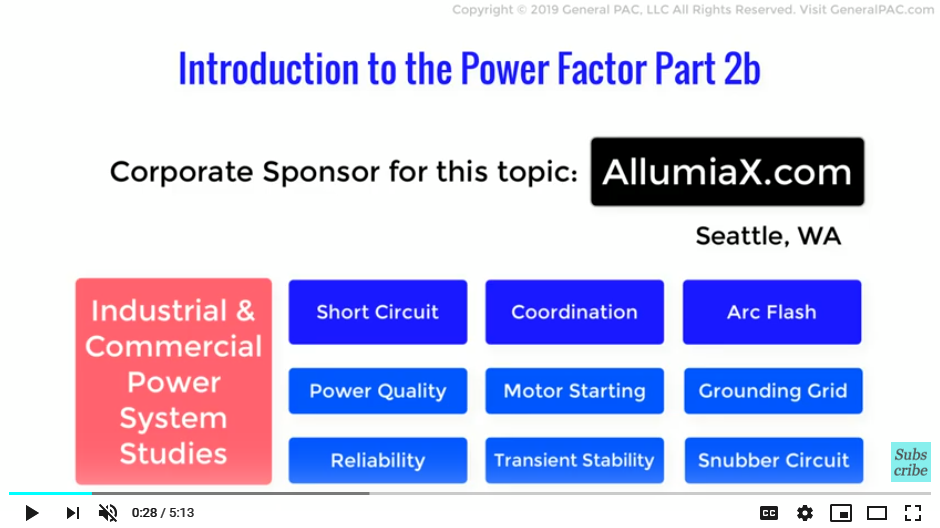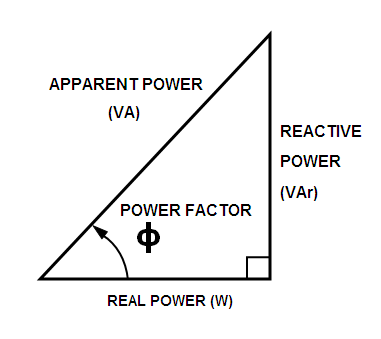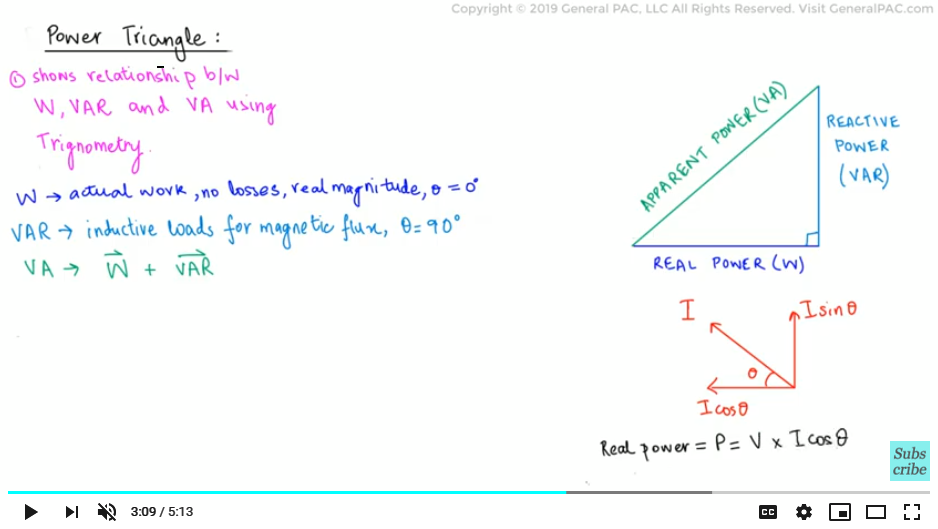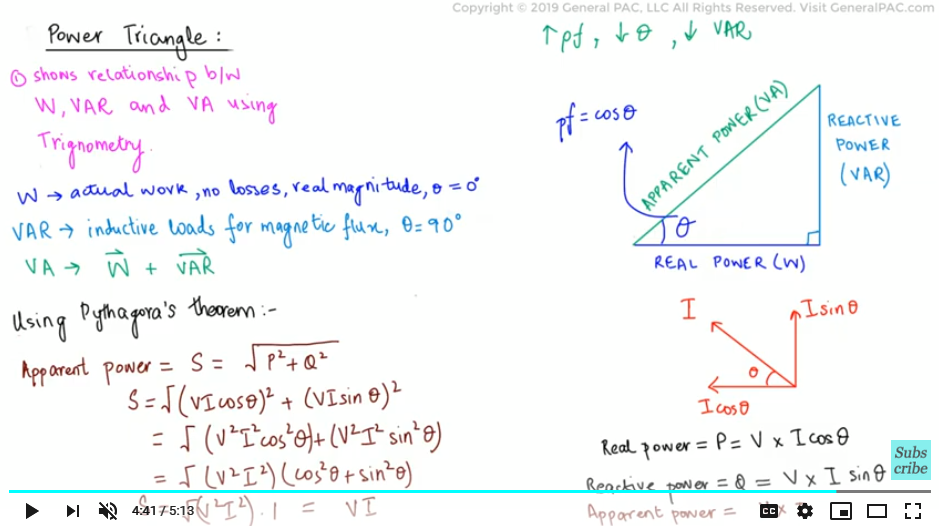This video was brought to you by GeneralPAC.com, making power systems Intuitive, Open and Free for Everyone, Everywhere. Consider subscribing and supporting through patreon.com/GeneralPAC. This is a mechanism for you to support us financially so we can continue making high quality power system video tutorials. Our corporate sponsor for this topic is AllumiaX.com from Seattle, Washington. Contact them for industrial and commercial power system studies.

Introduction to the Power Factor Part 2bIn the previous part, we had a look at a general overview of the concept behind power factor, and we used the phasor diagram to understand the relationship between the voltage and current in an AC circuit. In this part, we will use the previously established knowledge to construct a power triangle which will allow us to understand the relationships between the three types of power and the power factor.

Recalling previous parts and building upon the beer concept, the power triangle is simply a right angled triangle which shows the relationship between the three types of power using simple trigonometry. We shall first draw the real power component and establish the relationships from there. We know that real power is considered to be actual work done without any losses, therefore its magnitude is a real value in terms of watts and its angle is taken as zero due to no losses. Drawing this as a vector, we get the real power as a straight line. This indicates the Real Power.Next, we saw that reactive power is required by inductive loads for generating the magnetic flux. This reactive power causes the angle to shift between zero and 90 degrees, and is relative to the amount of magnetizing flux required by the inductive load. In a purely resistive circuit, this angle will be zero due to no magnetic flux requirement, but in a purely reactive load, this angle will shoot up to a maximum 90 degrees.

For our case, let us consider a purely reactive load, therefore we shall draw the reactive power as a perpendicular line to the real power.

Finally, as discussed in the previous part, the apparent power is the vector summation of the real and reactive power. Therefore, we shall add both components vectorially in the form of a hypotenuse. This indicates the apparent power. We can recall the fact that the current flowing in the AC circuit has two separate rectangular components in the form of the cosine and sine component.

If we want to model the components mathematically, the real power, denoted as P, will be the product of the voltage and the active component of the current. By multiplying with the cosine of the angle, we effectively get the horizontal or adjacent line of a right triangle.

Real Power=P=V x I x cos⁡θSimilarly, the reactive power, denoted as Q, will be the product of the voltage and the reactive component of the current. By multiplying with the sin of the angle, we effectively get the vertical or opposite line of a right triangle.

Reactive Power=Q=V x I x sin⁡θ

Next, we apply the well-known Pythagoras theorem to calculate the apparent power. Thus the apparent power, denoted as S, comes out to be the product of the magnitudes of the voltage and current. This effectively gives us the hypotenuse of a right triangle

Apparent Power=S=V x I

And finally we can see from the power triangle that there exists a phase angle between the apparent power and the active power. We know that the power factor is a measure of how effectively we are using electricity and equals the cosine of this phase angle. The greater the power factor, the lesser the phase angle and hence less reactive power is present in the circuit and vice versa.

Therefore, we want the phase angle to be as small as possible in order for us to get a higher power factor. However, that is not always the case as practical power systems consist of loads requiring reactive power as well.In part 3 we will look at some of the underlying reasons behind low power factors and the benefits which we can gain from improving it.

We hope, you have a continued interest in this topic and series as a student or professional. We also hope you find this content useful and enlightening. Please consider subscribing to GeneralPac.com or becoming a patron on patreon.com.

GeneralPAC.com. Making Power Systems Intuitive and free to everyone, everywhere.

#### Greetings from the GeneralPAC Team!

We make high-quality Power Systems Video Tutorials on complex topics that are free and open to everyone!  Thank you so much for supporting us through Patreon so we can continue doing good and valuable work.

What is Patreon and why do we use it?

Patreon is a fantastic portal that allows our fans and community to make monthly contribution (like Netflix subscription) so we can continue creating high-quality power systems video tutorials. In return, you get access to incredible perks like voting on future topics, getting your questions answered, access to VIP Q/A webinars with the creators of GeneralPAC, and much more! We THANK YOU for supporting us

Why do we need your support?

An incredible amount of time and effort is needed to develop high-quality video tutorials. Each video (Part 1 for example) takes approximately 10 hours to complete which includes learning the concept ourselves, brainstorming creative ways to teach and explain the concepts, writing the script, audio recording, video recording, and editing. It's no wonder why Hundreds-of-Thousands of people have watched, liked, subscribed, and left positive comments on Youtube channel. Your support truly makes all the difference.